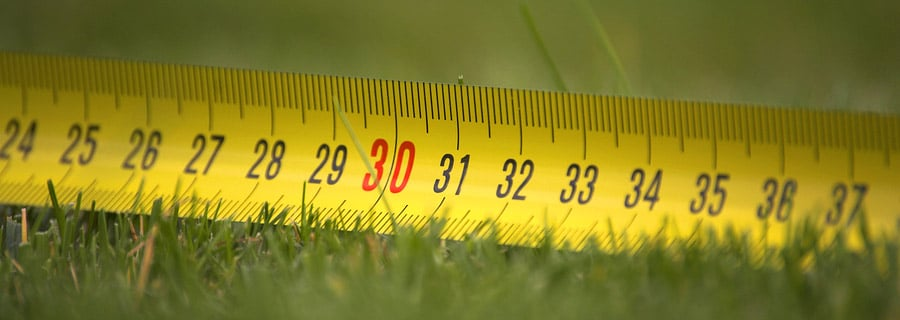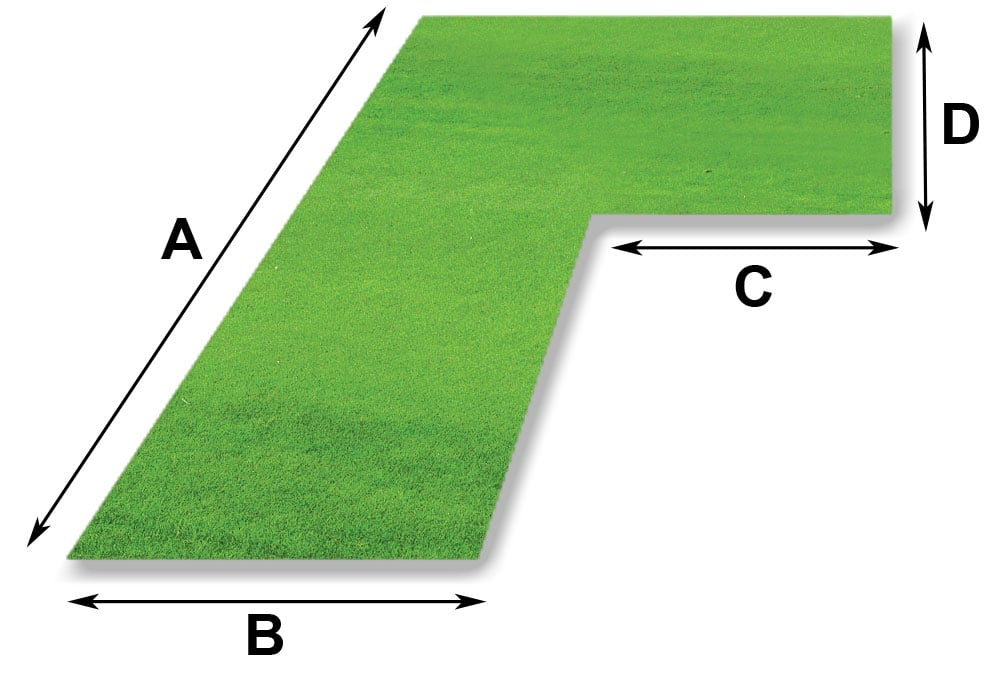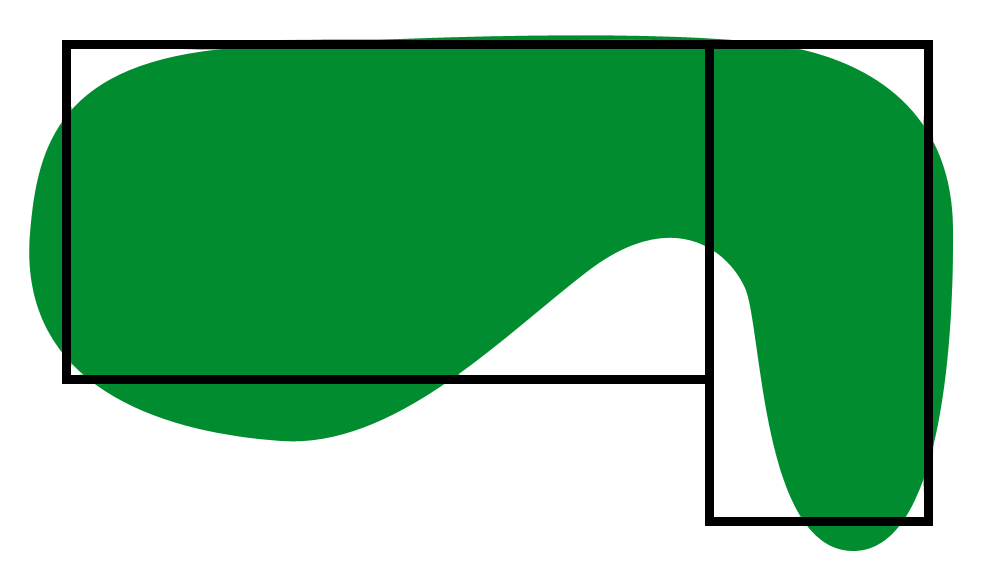# Guide to Measuring Your Lawn Ireland

Even the most awkwardly shaped lawns can be measured to a reasonable degree of accuracy if the correct approach is taken:

Square or rectangular lawn areas are easily calculated by multiplying the length by the width. Regardless of the units of measurement used, simply enter the details into our Emerald Lawn calculator and the required area will be determined.#### An example…

A=14m
B=2m
C=1.8m
D=5.5m

Area 1

A x B = 28m2

Area 2

C x D = 9.9m2

Total Area = 38m2Irregular shaped lawns should be sketched out on paper and then create as many squares or rectangles as necessary to cover your lawn sketch. Then each square or rectangle is easily calculated by multiplying the length by the width. Regardless of the units of measurement used, simply enter the details into our Emerald Lawn calculator and the required area will be determined.

Whatever the shape and size of your lawn, we strongly recommend that you purchase 5% more turf than you have calculated to cover for trimming losses. Our turf calculator automatically includes this margin.